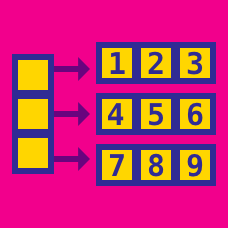Computer Science

# Linked Lists - Intermediate

Doubly linked lists are useful for more than just inserting and removing elements from the head and tail of the list. They can maintain a list of elements that allows for insertion and removal to the interior of the list

Given a node $$v$$ of a doubly linked list (which is currently followed by $$w$$), we want to insert a new node $$z$$ immediately after $$v$$. Concretely, we want to write a function insert(v) which takes a node, $$v$$, and inserts a new node after $$v$$. The result should be a list where $$v$$'s next points to $$z$$, $$z$$'s next points to $$w$$, $$w$$'s prev points to $$z$$, and $$z$$'s prev points to $$v$$.

If we execute these events in the wrong order, we can erase crucial information that will break the list. How should the following steps be ordered so that they correctly insert a node in-the middle of a doubly linked list?

• A Make $$z$$'s prev point to $$v$$.
• B Make $$v$$'s next point to $$z$$.
• C Make $$w$$'s prev point to $$z$$.
• D Make $$z$$'s next point to $$w$$.

Consider a circularly linked list. Which of the following methods below removes the node after the cursor? The cursor is a special node which allows us to have a place to start from if we ever need to traverse a circularly linked list.

  1 2 3 4 5 6 7 8 9 10 11 node A(node N){ Node oldNode = cursor.getNext(); if (oldNode == cursor) cursor = node.getNext() else{ cursor.setPrev(oldNode.getNext()); oldNode.setNext(null); } size --; return oldNode; } 

 1 2 3 4 5 node B(Node N){ Node oldNode = N oldNode.setNext(null) return oldNode; } 

  1 2 3 4 5 6 7 8 9 10 11 node C(Node N){ Node oldNode = cursor.getNext(); if (oldNode == cursor) cursor = null else{ cursor.setNext(oldNode.getNext()); oldNode.setNext(null); } size --; return oldNode; } 

  1 2 3 4 5 6 7 8 9 10 11 node D(Node N){ Node oldNode = N; if (oldNode == cursor) cursor = null else{ cursor.setNext(N); oldNode.setNext(null); } size --; return oldNode; } 

Given a singly linked list, write a program the shifts every node $$k$$ unit to the left.

For example

When 4$$\rightarrow$$6$$\rightarrow$$8$$\rightarrow$$9 is shifted $$2$$ units left it becomes 8$$\rightarrow$$9$$\rightarrow$$4$$\rightarrow$$6

What will the following linked list look like when each node is shifted $$4432897427834$$ to the left.

34$$\rightarrow$$17$$\rightarrow$$17$$\rightarrow$$74$$\rightarrow$$83$$\rightarrow$$59$$\rightarrow$$39

×

Problem Loading...

Note Loading...

Set Loading...